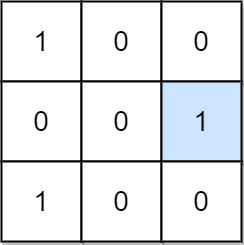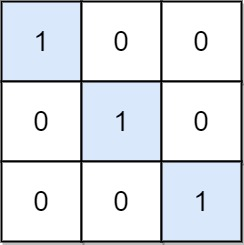1582. Special Positions in a Binary Matrix
Easy
635
23

Given an `m x n` binary matrix `mat`, return the number of special positions in `mat`.

A position `(i, j)` is called special if `mat[i][j] == 1` and all other elements in row `i` and column `j` are `0` (rows and columns are 0-indexed).

Example 1:```Input: mat = [[1,0,0],[0,0,1],[1,0,0]]
Output: 1
Explanation: (1, 2) is a special position because mat == 1 and all other elements in row 1 and column 2 are 0.
```

Example 2:```Input: mat = [[1,0,0],[0,1,0],[0,0,1]]
Output: 3
Explanation: (0, 0), (1, 1) and (2, 2) are special positions.
```

Constraints:

• `m == mat.length`
• `n == mat[i].length`
• `1 <= m, n <= 100`
• `mat[i][j]` is either `0` or `1`.
Accepted
41.6K
Submissions
63.9K
Acceptance Rate
65.1%

Seen this question in a real interview before?
1/4
Yes
No

Discussion (0)

Related Topics